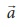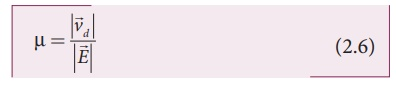Home | | Physics 12th Std | Drift velocity

# Drift velocity

The drift velocity is the average velocity acquired by the electrons inside the conductor when it is subjected to an electric field.

Drift velocity

In a conductor the charge carriers are free electrons. These electrons move freely through the conductor and collide repeatedly with the positive ions. If there is no electric field, the electrons move in random directions, so the directions of their velocities are also completely random direction. On an average, the number of electrons travelling in any direction will be equal to the number of electrons travelling in the opposite direction. As a result, there is no net flow of electrons in any direction and hence there will not be any current.

Suppose a potential difference is set across  the  conductor  by  connecting  a battery, an electric fieldis created in the conductor. This electric field exerts a force on the electrons, producing a current. The electric field accelerates the electrons, while ions scatter the electrons and change the direction of motion. Thus, we have zigzag paths of electrons. In addition to the zigzag motion due to the collisions, the electrons move slowly along the conductor in a direction opposite to that ofas shown in the Figure 2.4.Ions

Any material is made up of neutral atoms with equal number of electrons and protons. If the outermost electrons leave the atoms, they become free electrons and are responsible for electric current. The atoms after losing their outer most electrons will have more positive charges and hence are called positive ions. These ions will not move freely within the material like the free electrons. Hence the positive ions will not give rise to current.

This velocity is called drift velocityd . The drift velocity is the average velocity acquired by the electrons inside the conductor when it is subjected to an electric field. The average time between successive collisions is called the mean free time denoted by ¤ä. The accelerationexperienced by the electron in an electric fieldis given byHere ┬Á = e¤ä/m is the  mobility  of  the electron and it is defined as the magnitude of the drift velocity per unit electric field.The SI unit of mobility is m2/Vs.

### EXAMPLE 2.2

If an electric field of magnitude 570 N C-1, is applied in the copper wire, find the acceleration experienced by the electron.

### Solution:

E = 570 N C-1, e = 1.6 ├Ś 10-19 C, m = 9.11 ├Ś 10-31 kg and a = ?

F = ma = eE

a = eE/m = 570├Ś1 .6├Ś10Ôłĺ19/9 .11├Ś10-31

= 912 ├Ś10Ôłĺ19 ├Ś1031 / 9 .11

= 1.001 ├Ś 1014 m s-2

## Misconception

(i) There is a common misconception that the battery is the source of electrons. It is not true. When a battery is connected across the given wire, the electrons in the closed circuit resulting the current. Battery sets the potential difference (electrical energy) due to which these electrons in the conducting wire flow in a particular direction. The resulting electrical energy is used by electric bulb, electric fan etc. Similarly the electricity board is supplying the electrical energy to our home.

(ii) We often use the phrases like ÔÇścharging the battery in my mobileÔÇÖ and ÔÇśmy mobile phone battery has no chargeÔÇÖ etc. These sentences are not correct.When we say ÔÇśbattery has no chargeÔÇÖ, it means, that the battery has lost ability to provide energy or provide potential difference to the electrons in the circuit. When we say ÔÇśmobile is chargingÔÇÖ, it implies that the battery is receiving energy from AC power supply and not electrons.

Tags : Current Electricity , 12th Physics : Current Electricity
Study Material, Lecturing Notes, Assignment, Reference, Wiki description explanation, brief detail
12th Physics : Current Electricity : Drift velocity | Current Electricity